# Statistics Examples#

Some examples of how to compute statistics on M/EEG data with MNE.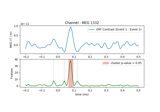Permutation F-test on sensor data with 1D cluster level

Permutation F-test on sensor data with 1D cluster level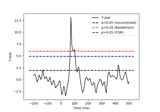FDR correction on T-test on sensor data

FDR correction on T-test on sensor data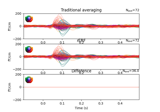Regression on continuous data (rER[P/F])

Regression on continuous data (rER[P/F])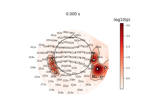Permutation T-test on sensor data

Permutation T-test on sensor data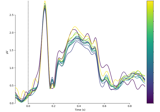Analysing continuous features with binning and regression in sensor space

Analysing continuous features with binning and regression in sensor space